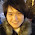### Json概述以及python對json的相關操作

JSON(JavaScript Object Notation) 是一種輕量級的數據交換格式。易於人閱讀和編寫。同時也易於機器解析和生成。它基於JavaScript Programming Language, Standard ECMA-262 3rd Edition - December 1999的一個子集。JSON採用完全獨立於語言的文本格式，但是也使用了類似於C語言家族的習慣（包括C, C++, C#, Java, JavaScript, Perl, Python等）。這些特性使JSON成為理想的數據交換語言。
JSON建構於兩種結構：
“名稱/值”對的集合（A collection of name/value pairs）。不同的語言中，它被理解為對象（object），紀錄（record），結構（struct），字典（dictionary），哈希表（hash table），有鍵列表（keyed list），或者關聯數組（associative array）。

jso官方說 ​​明參見：http://json.org/
Python操作json的標準api庫參考：http://docs.python.org/library/json.html

```
import  json

obj =  [[ 1 , 2 , 3 ], 123 , 123.123 , 'abc' ,{ 'key1' :( 1 , 2 , 3 ), 'key2' :( 4 , 5 , 6 )}]
encodedjson =  json.dumps(obj)
print  repr (obj)
print  encodedjson
```

[[1, 2, 3], 123, 123.123, 'abc', {'key2': (4, 5, 6), 'key1': (1, 2, 3)}]
[[1, 2, 3 ], 123, 123.123, "abc", {"key2": [4, 5, 6], "key1": [1, 2, 3]}]```
print  type (decodejson)
print  decodejson[ 4 ][ 'key1' ]
print  decodejson
```

[1, 2, 3]
[[1, 2, 3], 123, 123.123, u'abc', {u'key2': [4, 5, 6], u'key1': [1, 2, 3]}]json.dumps方法提供了很多好用的參數可供選擇，比較常用的有sort_keys（對dict對象進行排序，我們知道默認dict是無序存放的），separators，indent等參數。 排序功能使得存儲的數據更加有利於觀察，也使得對json輸出的對象進行比較，例如：
```
data1 =  { 'b' : 789 , 'c' : 456 , 'a' : 123 }
data2 =  { 'a' : 123 , 'b' : 789 , 'c' : 456 }
d1 =  json.dumps(data1,sort_keys = True )
d2 =  json.dumps(data2)
d3 =  json.dumps(data2,sort_keys = True )
print  d1
print  d2
print  d3
print  d1 = = d2
print  d1 = = d3
```

{"a": 123, "b": 789, "c": 456}
{"a": 123, "c": 456, "b": 789}
{"a": 123, "b": 789 , "c": 456}
False
True

indent參數是縮進的意思，它可以使得數據存儲的格式變得更加優雅。
```
data1 =  { 'b' : 789 , 'c' : 456 , 'a' : 123 }
d1 =  json.dumps(data1,sort_keys = True ,indent = 4 )
print  d1
```

```
{
"a": 123,
"b": 789,
"c": 456
}

```

```
print  'DATA:' , repr (data)
print  'repr(data) :' , len ( repr (data))
print  'dumps(data) :' , len (json.dumps(data))
print  'dumps(data, indent=2) :' , len (json.dumps(data, indent = 4 ))
print  'dumps(data, separators):' , len (json.dumps(data, separators = ( ',' , ':' )))

DATA: {'a': 123, 'c': 456, 'b': 789}
repr(data) : 30
dumps(data) : 30
dumps(data, indent=2) : 46
dumps(data, separators): 25
```

```
data =  { 'b' : 789 , 'c' : 456 ,( 1 , 2 ): 123 }
print  json.dumps(data,skipkeys = True )

{"c": 456, "b": 789}
```

json模塊不僅可以處理普通的python內置類型，也可以處理我們自定義的數據類型，而往往處理自定義的對像是很常用的。

```
class  Person( object ):
def  __init__( self ,name,age):
self .name =  name
self .age =  age
def  __repr__( self ):
return  'Person Object name : %s , age : %d'  %  ( self .name, self .age)
if  __name__   = =  '__main__' :
p =  Person( 'Peter' , 22 )
print  p
```

```
'''
Created on 2011-12-14
@author: Peter
'''
import  Person
import  json

p =  Person.Person( 'Peter' , 22 )

def  object2dict(obj):
#convert object to a dict
d =  {}
d[ '__class__' ] =  obj.__class__.__name__
d[ '__module__' ] =  obj.__module__
d.update(obj.__dict__)
return  d

def  dict2object(d):
#convert dict to object
if '__class__'  in  d:
class_name =  d.pop( '__class__' )
module_name =  d.pop( '__module__' )
module =  __import__ (module_name)
class_  =  getattr (module,class_name)
args =  dict ((key.encode( 'ascii' ), value) for  key, value in  d.items()) #get args
inst =  class_ ( * * args) #create new instance
else :
inst =  d
return  inst

d =  object2dict(p)
print  d
#{'age': 22, '__module__': 'Person', '__class__': 'Person', 'name': 'Peter'}

o =  dict2object(d)
print  type (o),o
# Person Object name : Peter , age : 22

dump =  json.dumps(p,default = object2dict)
print  dump
#{"age": 22, "__module__": "Person", "__class__": "Person", "name": "Peter"}

#Person Object name : Peter , age : 22
```

JSONEncoder類負責編碼，主要是通過其default函數進行轉化，我們可以override該方法。同理對於JSONDecoder。
```
'''
Created on 2011-12-14
@author: Peter
'''
import  Person
import  json

p =  Person.Person( 'Peter' , 22 )

class  MyEncoder(json.JSONEncoder):
def  default( self ,obj):
#convert object to a dict
d =  {}
d[ '__class__' ] =  obj.__class__.__name__
d[ '__module__' ] =  obj.__module__
d.update(obj.__dict__)
return  d

class  MyDecoder(json.JSONDecoder):
def  __init__( self ):
json.JSONDecoder.__init__( self ,object_hook = self .dict2object)
def  dict2object( self ,d):
#convert dict to object
if '__class__'  in  d:
class_name =  d.pop( '__class__' )
module_name =  d.pop( '__module__' )
module =  __import__ (module_name)
class_  =  getattr (module,class_name)
args =  dict ((key.encode( 'ascii' ), value) for  key, value in  d.items()) #get args
inst =  class_ ( * * args) #create new instance
else :
inst =  d
return  inst

d =  MyEncoder().encode(p)
o =   MyDecoder().decode(d)

print  d
print  type (o), o
```

### 留言

1.您好，
請問如果遇到object本身是list的情況怎麼辦?
在Encoder那邊，我發現可以用:
class MyEncoder(json.JSONEncoder):
def default( self ,obj):
#convert object to a dict
d = {}
if isinstance(object_, list):
return json.dumps(object_, default=self.default)
else:
d[ '__class__' ] = obj.__class__.__name__
d[ '__module__' ] = obj.__module__
d.update(obj.__dict__)
return d

但是Decoder的部分怎麼辦? 有勞您解答了~~

1.reference: https://stackoverflow.com/questions/26033239/list-of-objects-to-json-with-python

2.感謝文章，易讀易懂，多謝啦!!!